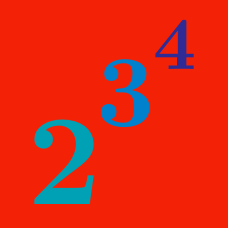Algebra

# Rules of Exponents - Tower Rule

Evaluate $\Large 3^{2^3}.$

Evaluate

$\huge 4^{3^{2^{1^{0}}}}.$

$\Large P = \frac{4^{3^2}}{2^{3^4}}$

Which of the following is correct about the above number $$P?$$

$\Huge 2^{2^{2^{2^2}}} \qquad 3^{3^{3^3}} \qquad 10^{10^{10}}$

Which of the above is the largest?

$\Large 10^{10^{10}}$

How many digits does the above number have?

×

Problem Loading...

Note Loading...

Set Loading...